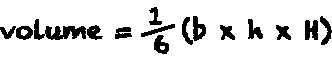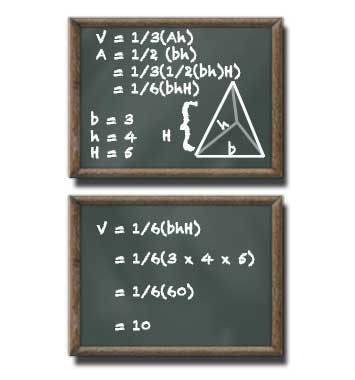# Right-Triangular Pyramid Volume Calculator

## Right-Triangular Pyramid Volume

#### Volume of a Pyramid with Right-Triangular Base

Result:
0.5base (b) units
height (h) decimals
Height (H)

A right-angle triangular pyramid with a base of 3 units a triangle height of 4 units and a pyramid height of 5 units has a volume of 10 cubic units.

This calculator and more easy to use calculators waiting at www.KylesCalculators.com### Calculating the Volume of a Right-Triangular Pyramid:

A right-triangular pyramid is a three dimensional shape with a right-angle triangle at its base extruding up to a single point. The volume of a right-triangular pyramid can be calculated according to the following formula:

V = 1/3(Ah)
where A = 1/2(bh) (standard right-triangle)
so : V = 1/6(bhH)

Explanation: The general formula used to find the volume of a pyramid is one-third times area of the base times height of the pyramid. Because it is known that this pyramid has a right-triangle at its base the formula for a right-triangle is used to calculate the area. The formula one-half base times height is inserted into the volume formula in the place of area. After multiplying out the formula now reads one-sixth time base of triangle (b) times height of triangle (h) times height of pyramid (H).

Below is an example of how to find the volume of this type of triangular pyramid. In this example the triangle at the base of the pyramid must have a ninety degree (right) angle. Lets say the triangle has a base of 3 meters, a height of 4 meters and that the pyramid is 5 meters tall:

• V = 1/6(bhH)
• = 1/6 (3 x 4 x 5)
• = 1/6 (60)
• = 10 meters